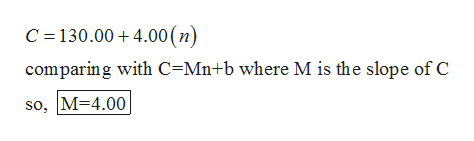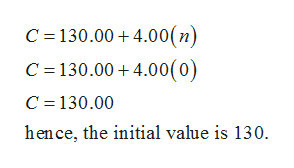XContent90%Ohttps://elearning.kctcs.edu/ultra/courses/ 493523760 1/cl/outlineAn elementary school is taking a busload of children to a science fair. It costs \$130.00 to drive the bus to the fair and back, and the school pays each student's \$4.00 admission fee.(a) Use a formula to express the total cost C, in dollars, of the science fair trip as a linear function of the number n of children who make the trip.c 130.00 4.00 (n)(b) Identify the slope and initial value of C.slopeinitial valueExplain in practical terms what these values mean.and it is the cost of taking the busThe initial value isThe slope indicates that for each additional child we take on the trip the total cost increases byitself to the fair.(c) Explain in practical terms what C(9) means.C(9) represents the total cost(in dollars) of the science fair trip if 9 children make the trip.Calculate C(9).\$10:37 PM9/29/2019ype here to searchAhp XXContentX90%https://elearning.kctcs.edu/ultra/courses/_493523760 1/cl/outlineaS a llnedl lunctionT OI une riuIber or CIaren WTIO make Ue uip.QOllar s, olUie science Tal upuie toldl COSL C, I(a) use a TOMmula tO expressC 130.00+4.00 (n)(b) Identify the slope and initial value of C.slopeinitial valueExplain in practical terms what these values mean.and it is the cost of taking the busThe initial value isThe slope indicates that for each additional child we take on the trip the total cost increases byitself to the fair.(c) Explain in practical terms what C(9) means.(in dollars) of the science fair trip if 9 children make the trip.C(9) represents the total costCalculate C(9)(d) Solve the equation C(n) - 178 for n.Explain what the answer you get represents.to spend.178 is the number of students we can take if there is \$The solution of the equation 4n + 130Save Assignment ProgressSubmit Assignment10:37 PMx 9/29/20198Type here to search

Question

what are the blanks

Step 1

From the given information:

C is taken as total cost and n is taken to be number of children and this is defined as:

Step 2

By comparing the above equation with c=mx+bhelp_outlineImage TranscriptioncloseC 130.00 4.00(n) comparing with C Mn+b where M is the slope of C so, M 4.00 fullscreen
Step 3

Now, to find the initia...help_outlineImage TranscriptioncloseC 130.00 4.00(n) C 130.00 4.00(0) C 130.00 hence, the initial value is 130. fullscreen

Want to see the full answer?

See Solution

Want to see this answer and more?

Our solutions are written by experts, many with advanced degrees, and available 24/7

See Solution
Tagged in

Other